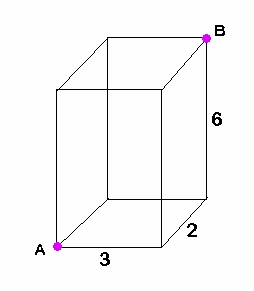# ACT, Geometry – diagonal of a rectangular prism

Q: What is the (straight-line) distance between points A and B on the figure below?Explanation:  We are finding the length of the green segment below:This requires the three-dimensional version of the distance formula:

There is another way to do this, using a progression of two right triangles, with the green segment being the hypotenuse of the second triangle, but I find the above formula much simpler.  It is just an extension of the ‘usual’ (2-dimensional) distance formula we are so familiar with from Algebra and Geometry.

BUT if you want an even easier way, and I know you do, use my TI-84 Plus program ‘DIST3D’.Lorem ipsum dolor sit amet, consectetur adipisicing elit, sed do eiusmod tempor incididunt ut labore et dolore magna aliqua. Ut enim ad minim veniam, quis nostrud exercitation ullamco laboris nisi ut aliquip ex ea commodo consequat.

# Geometry – Volume

Q: What is the maximum number of boxes with dimensions 4”x5”x8” that can be packed into one large box with dimensions 20”x20”x24”?

Explanation: First, decide that a whole number of small boxes will entirely fill the large box.  To do this, look at the dimensions and match them.  4″ fits into 20″ five times.  5″ fits into 20″ four times.  8″ fits into 24″ three times.  That means, you’ll get five rows of small boxes, four boxes per row, and three layers deep.  So, the quickest way to get the answer is to multiply 5x4x3 = 60.

BUT if you don’t notice that, just do (large volume)/(small volume).

Large volume = 20x20x24 = 9600

Small volume = 4x5x8 = 160

(large volume)/(small volume) = 9600/160 = 60